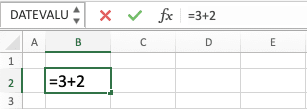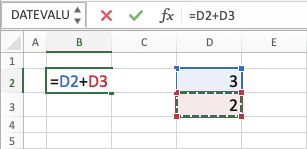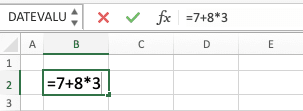How to Do Excel Calculations - Compute Expert

# How to Do Excel Calculations

Home >> Excel Tutorials from Compute Expert >> How to Do Excel Calculations

The calculation process is an important thing to master when you work in excel. After all, some, if not most, data we process in the software are quantitative numbers. If you cannot perform calculations, then you won’t get optimal results from what you work on in excel.

Therefore, to help with that, we will discuss the basics of how to do numbers calculation in excel here. If you need a more specific calculation tutorial, then you can click one of our tutorial links at the bottom.

## Ways to Calculate in Excel

There are many methods you can utilize to calculate numbers in excel. However, two of the most common methods people use are by writing a manual formula or by using excel functions.

Both methods require you to write an equal symbol (=) first as the sign that you want to write a formula. The first method, writing a manual formula, uses calculation symbols to perform the calculation process.

We can use this first method to do basic calculations such as addition, subtraction, multiplication, division, power, and root. All of them have their own symbol (we will discuss this further in the next part of this tutorial) and you must type the appropriate symbol to do your calculation.

Here is an implementation example of writing a manual formula to do a calculation in excel. In this example, we write a formula to add 3 and 2.As you can see there, we write an equal symbol, the numbers we want to add, and the addition symbol (a plus sign). After we write that, we press enter and we will get our addition result.Quite simple, isn’t it? Do that for other calculations with their symbols and you can do your calculation in excel smoothly.

But, what about the second method, by using the excel functions?

Well, excel provides us with many functions that we can use to process our data automatically. Some of those functions can help us to perform calculation processes in excel. We just need to write the function name and provides its correct inputs in a bracket to do the calculation.

A different calculation process requires a different excel function. Here is an implementation example of the excel function to add the numbers we have added previously using the manual formula. In this example, we use the excel numbers addition function, SUM.We write in the cell using the pattern we discussed just now. We write an equal symbol, the function name (SUM), and the inputs that this SUM function needs (which are the numbers we want to add).

We press enter after doing that and we will get our calculation result.Those two methods aren’t too hard to do, right? Besides typing the numbers directly, you can also refer to cell coordinates if your numbers are there like below.In the example, D2 and D3 we write in our formula are the cell coordinates of the numbers we add. D is the column letter while 2 and 3 are the row numbers. As you can see, we can get the same addition result by referring to our numbers cell coordinates instead.

Just remember to use the correct symbol and excel function so you can get your calculation result. If you need to know what symbol and function to use for a calculation type, see our tutorials at the bottom!

## Calculation Symbols in Excel

What are the symbols we can use to calculate our numbers in excel? Here is a table that contains the information of those symbols and what calculation they can perform.

SymbolCalculationFormula Writing ExampleExplanation
- (dash sign)Subtraction=3-2Subtract three with two
* (star symbol)Multiplication=3*2Multiply three with two
/ (slash sign)Division=3/2Divide three with two
^ (caret symbol)Power=3^2Power three by two
^ (caret symbol)Root=3^(1/2)Square root three

## Order of Calculation in Excel

The calculation process in excel follows a standard calculation order. This order means the calculation type in the earlier order will normally run first than the one in the later order.

You should consider this order when you write a calculation in excel so you can get the correct result. For reference, here is a table that summarizes the calculation order in excel.

OrderCalculation
1Negation (add a minus in front of a number to convert it to a negative/positive number)
2Percent
3Power/root
4Multiplication/division

## Change the Calculation Order in Excel

We have learned about the calculation order and that the calculation in the earlier order will normally run first. But what if we want to, for example, add numbers first before we do the multiplication in one formula writing? Can we do that?

We can do that by using a bracket on the calculation we want to run first. By doing this, we can change the calculation order in our formula writing to the way we prefer.

For a better understanding of this, let’s take a look at the example below. Let’s say we want to add some numbers before we multiply the addition result with another number. If we write our calculation without a bracket, then this will happen.That formula writing will multiply 8 with 3 first before it adds the result with 7. As a result, we get 31 there (the multiplication of 8 and 3 equals 24 and the addition of 24 and 7 equals 31).

However, if we put a bracket in the 7 and 8 addition, then we can add them first before the multiplication.See that the result changes to 45? That is because excel runs the addition process of 7 and 8 first before it multiplies the result with 3 (the addition of 7 and 8 equals 15 and the multiplication of 15 and 3 equals 45). We change the calculation order to the way we want by using the bracket on our addition writing!

Use brackets wisely and you should get the correct result from your calculation formula writing. This is important especially if you write a long calculation formula that has several calculations running.

## Excel Calculation Tutorials

Now that you have learned the basics of excel calculation, you may want to learn more about a specific calculation type. For that, you can learn the ones you need by clicking on our tutorial links below!

These tutorials will give you a complete overview of how to perform a specific calculation type in excel. You will learn about the formula writings, the functions/features you can use for particular calculation situations, and more. There is also a simple exercise in each tutorial so you can understand the tutorial topic more practically.

Currently, there are 16 excel calculation tutorials here. We will add more in the future if we see there are many demands for a new, interesting excel calculation topic!

Click one of them right away to begin your learning!

Get updated excel info from Compute Expert by registering your email. It's free!